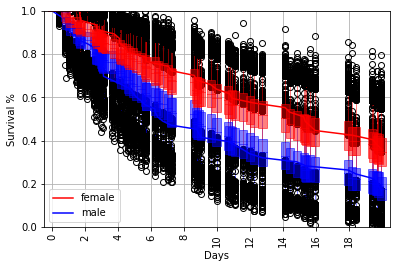In :
%load_ext autoreload
%matplotlib inline
import random
random.seed(1100038344)
import survivalstan
import numpy as np
import pandas as pd
from stancache import stancache
from matplotlib import pyplot as plt

INFO:stancache.seed:Setting seed to 1245502385


## The model¶

This style of modeling is often called the "piecewise exponential model", or PEM. It is the simplest case where we estimate the hazard of an event occurring in a time period as the outcome, rather than estimating the survival (ie, time to event) as the outcome.

Recall that, in the context of survival modeling, we have two models:

1. A model for Survival ($S$), ie the probability of surviving to time $t$:

$$S(t)=Pr(Y > t)$$

2. A model for the instantaneous hazard $\lambda$, ie the probability of a failure event occuring in the interval [$t$, $t+\delta t$], given survival to time $t$:

$$\lambda(t) = \lim_{\delta t \rightarrow 0 } \; \frac{Pr( t \le Y \le t + \delta t | Y > t)}{\delta t}$$

By definition, these two are related to one another by the following equation:

$$\lambda(t) = \frac{-S'(t)}{S(t)}$$

Solving this, yields the following:

$$S(t) = \exp\left( -\int_0^t \lambda(z) dz \right)$$

This model is called the piecewise exponential model because of this relationship between the Survival and hazard functions. It's piecewise because we are not estimating the instantaneous hazard; we are instead breaking time periods up into pieces and estimating the hazard for each piece.

There are several variations on the PEM model implemented in survivalstan. In this notebook, we are exploring just one of them.

### A note about data formatting¶

When we model Survival, we typically operate on data in time-to-event form. In this form, we have one record per Subject (ie, per patient). Each record contains [event_status, time_to_event] as the outcome. This data format is sometimes called per-subject.

When we model the hazard by comparison, we typically operate on data that are transformed to include one record per Subject per time_period. This is called per-timepoint or long form.

All other things being equal, a model for Survival will typically estimate more efficiently (faster & smaller memory footprint) than one for hazard simply because the data are larger in the per-timepoint form than the per-subject form. The benefit of the hazard models is increased flexibility in terms of specifying the baseline hazard, time-varying effects, and introducing time-varying covariates.

In this example, we are demonstrating use of the standard PEM survival model, which uses data in long form. The stan code expects to recieve data in this structure.

## Stan code for the model¶

This model is provided in survivalstan.models.pem_survival_model. Let's take a look at the stan code.

In :
print(survivalstan.models.pem_survival_model)

/*  Variable naming:
// dimensions
N          = total number of observations (length of data)
S          = number of sample ids
T          = max timepoint (number of timepoint ids)
M          = number of covariates

// main data matrix (per observed timepoint*record)
s          = sample id for each obs
t          = timepoint id for each obs
event      = integer indicating if there was an event at time t for sample s
x          = matrix of real-valued covariates at time t for sample n [N, X]

// timepoint-specific data (per timepoint, ordered by timepoint id)
t_obs      = observed time since origin for each timepoint id (end of period)
t_dur      = duration of each timepoint period (first diff of t_obs)

*/
// Jacqueline Buros Novik <[email protected]>

data {
// dimensions
int<lower=1> N;
int<lower=1> S;
int<lower=1> T;
int<lower=0> M;

// data matrix
int<lower=1, upper=N> s[N];     // sample id
int<lower=1, upper=T> t[N];     // timepoint id
int<lower=0, upper=1> event[N]; // 1: event, 0:censor
matrix[N, M] x;                 // explanatory vars

// timepoint data
vector<lower=0>[T] t_obs;
vector<lower=0>[T] t_dur;
}
transformed data {
vector[T] log_t_dur;  // log-duration for each timepoint
int n_trans[S, T];

log_t_dur = log(t_dur);

// n_trans used to map each sample*timepoint to n (used in gen quantities)
// map each patient/timepoint combination to n values
for (n in 1:N) {
n_trans[s[n], t[n]] = n;
}

// fill in missing values with n for max t for that patient
// ie assume "last observed" state applies forward (may be problematic for TVC)
// this allows us to predict failure times >= observed survival times
for (samp in 1:S) {
int last_value;
last_value = 0;
for (tp in 1:T) {
// manual says ints are initialized to neg values
// so <=0 is a shorthand for "unassigned"
if (n_trans[samp, tp] <= 0 && last_value != 0) {
n_trans[samp, tp] = last_value;
} else {
last_value = n_trans[samp, tp];
}
}
}
}
parameters {
vector[T] log_baseline_raw; // unstructured baseline hazard for each timepoint t
vector[M] beta;         // beta for each covariate
real<lower=0> baseline_sigma;
real log_baseline_mu;
}
transformed parameters {
vector[N] log_hazard;
vector[T] log_baseline;     // unstructured baseline hazard for each timepoint t

log_baseline = log_baseline_mu + log_baseline_raw + log_t_dur;

for (n in 1:N) {
log_hazard[n] = log_baseline[t[n]] + x[n,]*beta;
}
}
model {
beta ~ cauchy(0, 2);
event ~ poisson_log(log_hazard);
log_baseline_mu ~ normal(0, 1);
baseline_sigma ~ normal(0, 1);
log_baseline_raw ~ normal(0, baseline_sigma);
}
generated quantities {
real log_lik[N];
vector[T] baseline;
real y_hat_time[S];      // predicted failure time for each sample
int y_hat_event[S];      // predicted event (0:censor, 1:event)

// compute raw baseline hazard, for summary/plotting
baseline = exp(log_baseline_mu + log_baseline_raw);

// prepare log_lik for loo-psis
for (n in 1:N) {
log_lik[n] = poisson_log_log(event[n], log_hazard[n]);
}

// posterior predicted values
for (samp in 1:S) {
int sample_alive;
sample_alive = 1;
for (tp in 1:T) {
if (sample_alive == 1) {
int n;
int pred_y;
real log_haz;

// determine predicted value of this sample's hazard
n = n_trans[samp, tp];
log_haz = log_baseline[tp] + x[n,] * beta;

// now, make posterior prediction of an event at this tp
if (log_haz < log(pow(2, 30)))
pred_y = poisson_log_rng(log_haz);
else
pred_y = 9;

// summarize survival time (observed) for this pt
if (pred_y >= 1) {
// mark this patient as ineligible for future tps
// note: deliberately treat 9s as events
sample_alive = 0;
y_hat_time[samp] = t_obs[tp];
y_hat_event[samp] = 1;
}

}
} // end per-timepoint loop

// if patient still alive at max
if (sample_alive == 1) {
y_hat_time[samp] = t_obs[T];
y_hat_event[samp] = 0;
}
} // end per-sample loop
}



## Simulate survival data¶

In order to demonstrate the use of this model, we will first simulate some survival data using survivalstan.sim.sim_data_exp_correlated. As the name implies, this function simulates data assuming a constant hazard throughout the follow-up time period, which is consistent with the Exponential survival function.

This function includes two simulated covariates by default (age and sex). We also simulate a situation where hazard is a function of the simulated value for sex.

We also center the age variable since this will make it easier to interpret estimates of the baseline hazard.

In :
d = stancache.cached(
survivalstan.sim.sim_data_exp_correlated,
N=100,
censor_time=20,
rate_form='1 + sex',
rate_coefs=[-3, 0.5],
)
d['age_centered'] = d['age'] - d['age'].mean()

INFO:stancache.stancache:sim_data_exp_correlated: cache_filename set to sim_data_exp_correlated.cached.N_100.censor_time_20.rate_coefs_54462717316.rate_form_1 + sex.pkl


Aside: In order to make this a more reproducible example, this code is using a file-caching function stancache.cached to wrap a function call to survivalstan.sim.sim_data_exp_correlated.

## Explore simulated data¶

Here is what these data look like - this is per-subject or time-to-event form:

In :
d.head()

Out:
sex age rate true_t t event index age_centered
0 male 54 0.082085 1.013855 1.013855 True 0 -1.12
1 male 39 0.082085 4.890597 4.890597 True 1 -16.12
2 female 45 0.049787 4.093404 4.093404 True 2 -10.12
3 female 43 0.049787 7.036226 7.036226 True 3 -12.12
4 female 57 0.049787 5.712299 5.712299 True 4 1.88

It's not that obvious from the field names, but in this example "subjects" are indexed by the field index.

We can plot these data using lifelines, or the rudimentary plotting functions provided by survivalstan.

In :
survivalstan.utils.plot_observed_survival(df=d[d['sex']=='female'], event_col='event', time_col='t', label='female')
survivalstan.utils.plot_observed_survival(df=d[d['sex']=='male'], event_col='event', time_col='t', label='male')
plt.legend()

Out:
<matplotlib.legend.Legend at 0x7efde84c26d8>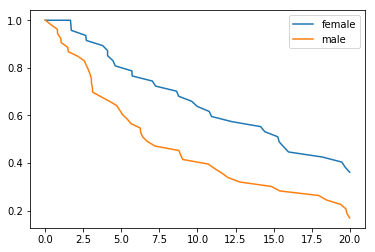## Transform to long or per-timepoint form¶

Finally, since this is a PEM model, we transform our data to long or per-timepoint form.

In :
dlong = stancache.cached(
survivalstan.prep_data_long_surv,
df=d, event_col='event', time_col='t'
)

INFO:stancache.stancache:prep_data_long_surv: cache_filename set to prep_data_long_surv.cached.df_14209590808.event_col_event.time_col_t.pkl


We now have one record per timepoint (distinct values of end_time) per subject (index, in the original data frame).

In :
dlong.query('index == 1').sort_values('end_time')

Out:
sex age rate true_t t event index age_centered end_time end_failure
148 male 39 0.082085 4.890597 4.890597 True 1 -16.12 0.009787 False
140 male 39 0.082085 4.890597 4.890597 True 1 -16.12 0.377535 False
147 male 39 0.082085 4.890597 4.890597 True 1 -16.12 0.791192 False
133 male 39 0.082085 4.890597 4.890597 True 1 -16.12 0.808987 False
75 male 39 0.082085 4.890597 4.890597 True 1 -16.12 1.013855 False
128 male 39 0.082085 4.890597 4.890597 True 1 -16.12 1.052508 False
106 male 39 0.082085 4.890597 4.890597 True 1 -16.12 1.467963 False
102 male 39 0.082085 4.890597 4.890597 True 1 -16.12 1.517398 False
118 male 39 0.082085 4.890597 4.890597 True 1 -16.12 1.653389 False
123 male 39 0.082085 4.890597 4.890597 True 1 -16.12 1.684769 False
119 male 39 0.082085 4.890597 4.890597 True 1 -16.12 1.713038 False
104 male 39 0.082085 4.890597 4.890597 True 1 -16.12 2.125944 False
103 male 39 0.082085 4.890597 4.890597 True 1 -16.12 2.558112 False
135 male 39 0.082085 4.890597 4.890597 True 1 -16.12 2.656621 False
92 male 39 0.082085 4.890597 4.890597 True 1 -16.12 2.692360 False
138 male 39 0.082085 4.890597 4.890597 True 1 -16.12 2.701946 False
95 male 39 0.082085 4.890597 4.890597 True 1 -16.12 2.829331 False
87 male 39 0.082085 4.890597 4.890597 True 1 -16.12 2.942428 False
108 male 39 0.082085 4.890597 4.890597 True 1 -16.12 3.025977 False
125 male 39 0.082085 4.890597 4.890597 True 1 -16.12 3.034875 False
93 male 39 0.082085 4.890597 4.890597 True 1 -16.12 3.095593 False
129 male 39 0.082085 4.890597 4.890597 True 1 -16.12 3.111741 False
139 male 39 0.082085 4.890597 4.890597 True 1 -16.12 3.641794 False
117 male 39 0.082085 4.890597 4.890597 True 1 -16.12 3.771836 False
83 male 39 0.082085 4.890597 4.890597 True 1 -16.12 4.092917 False
77 male 39 0.082085 4.890597 4.890597 True 1 -16.12 4.093404 False
145 male 39 0.082085 4.890597 4.890597 True 1 -16.12 4.200436 False
126 male 39 0.082085 4.890597 4.890597 True 1 -16.12 4.451497 False
89 male 39 0.082085 4.890597 4.890597 True 1 -16.12 4.591072 False
81 male 39 0.082085 4.890597 4.890597 True 1 -16.12 4.694461 False
76 male 39 0.082085 4.890597 4.890597 True 1 -16.12 4.890597 True

## Fit stan model¶

Now, we are ready to fit our model using survivalstan.fit_stan_survival_model.

We pass a few parameters to the fit function, many of which are required. See ?survivalstan.fit_stan_survival_model for details.

Similar to what we did above, we are asking survivalstan to cache this model fit object. See stancache for more details on how this works. Also, if you didn't want to use the cache, you could omit the parameter FIT_FUN and survivalstan would use the standard pystan functionality.

In :
testfit = survivalstan.fit_stan_survival_model(
model_cohort = 'test model',
model_code = survivalstan.models.pem_survival_model,
df = dlong,
sample_col = 'index',
timepoint_end_col = 'end_time',
event_col = 'end_failure',
formula = '~ age_centered + sex',
iter = 5000,
chains = 4,
seed = 9001,
FIT_FUN = stancache.cached_stan_fit,
)

/home/jacki/projects/survivalstan/survivalstan/survivalstan.py:368: FutureWarning: Method .as_matrix will be removed in a future version. Use .values instead.
'x': self.x_df.as_matrix(),
INFO:stancache.stancache:Step 1: Get compiled model code, possibly from cache
INFO:stancache.stancache:StanModel: cache_filename set to anon_model.cython_0_29_2.model_code_6711018461227478976.pystan_2_18_1_0.stanmodel.pkl
INFO:stancache.stancache:Step 2: Get posterior draws from model, possibly from cache
INFO:stancache.stancache:sampling: cache_filename set to anon_model.cython_0_29_2.model_code_6711018461227478976.pystan_2_18_1_0.stanfit.chains_4.data_75284643319.iter_5000.seed_9001.pkl


## Superficial review of convergence¶

We will note here some top-level summaries of posterior draws -- this is a minimal example so it's unlikely that this model converged very well.

In practice, you would want to do a lot more investigation of convergence issues, etc. For now the goal is to demonstrate the functionalities available here.

We can summarize posterior estimates for a single parameter, (e.g. the built-in Stan parameter lp__):

In :
survivalstan.utils.print_stan_summary([testfit], pars='lp__')

            mean    se_mean         sd        2.5%         50%       97.5%      Rhat
lp__ -343.716157  29.967469  84.346802 -428.904008 -371.820577 -101.853053  1.943707


Or, for sets of parameters with the same name:

In :
survivalstan.utils.print_stan_summary([testfit], pars='log_baseline_raw')

                          mean   se_mean        sd      2.5%       50%     97.5%      Rhat
log_baseline_raw   0.127908  0.011206  0.390646 -0.574587  0.045060  1.112140  1.009859
log_baseline_raw  -0.180508  0.023764  0.347081 -1.042441 -0.093197  0.359572  1.024901
log_baseline_raw  -0.204398  0.026817  0.353415 -1.078168 -0.110903  0.339344  1.031154
log_baseline_raw   0.111204  0.010062  0.374736 -0.587863  0.032338  1.035699  1.008499
log_baseline_raw  -0.076325  0.005433  0.334775 -0.865588 -0.019305  0.555003  1.004544
log_baseline_raw   0.088543  0.005141  0.366412 -0.627267  0.028007  0.930208  1.004422
log_baseline_raw  -0.192198  0.026126  0.345796 -1.023044 -0.100468  0.334006  1.028990
log_baseline_raw   0.077545  0.005527  0.362746 -0.625327  0.021046  0.914410  1.003724
log_baseline_raw  -0.007915  0.002938  0.340064 -0.792517  0.000030  0.720585  0.999816
log_baseline_raw  0.102421  0.008460  0.372675 -0.585478  0.031353  1.009513  1.006293
log_baseline_raw  0.105546  0.008672  0.375497 -0.595675  0.028599  1.018843  1.007367
log_baseline_raw -0.179333  0.024334  0.341492 -1.029340 -0.095580  0.361123  1.024614
log_baseline_raw -0.189801  0.025664  0.351737 -1.063312 -0.098743  0.337103  1.027370
log_baseline_raw  0.030662  0.002810  0.348627 -0.716552  0.007330  0.795914  1.000677
log_baseline_raw  0.099584  0.007343  0.363191 -0.583793  0.027420  0.982254  1.006853
log_baseline_raw  0.127511  0.013778  0.396442 -0.575169  0.040235  1.085049  1.010791
log_baseline_raw  0.008104  0.002762  0.334577 -0.717536  0.001003  0.718622  0.999951
log_baseline_raw  0.017583  0.002839  0.348238 -0.739866  0.004059  0.786641  1.000018
log_baseline_raw  0.051770  0.003351  0.346987 -0.643967  0.012692  0.845073  1.001971
log_baseline_raw  0.129739  0.012444  0.392641 -0.551537  0.043589  1.088190  1.010026
log_baseline_raw  0.070802  0.003980  0.362372 -0.631452  0.017359  0.915291  1.003119
log_baseline_raw  0.120558  0.011412  0.401495 -0.612580  0.037298  1.107091  1.008950
log_baseline_raw -0.200919  0.026878  0.348461 -1.059523 -0.105612  0.319076  1.031085
log_baseline_raw  0.011504  0.002747  0.333063 -0.706409  0.002467  0.729663  0.999909
log_baseline_raw -0.100401  0.008833  0.342553 -0.927089 -0.029254  0.510422  1.007842
log_baseline_raw  0.145832  0.013621  0.409583 -0.548009  0.052751  1.148941  1.012278
log_baseline_raw  0.036857  0.003076  0.347189 -0.713045  0.009555  0.804570  1.000600
log_baseline_raw -0.050060  0.003738  0.332868 -0.821535 -0.009052  0.584618  1.002173
log_baseline_raw  0.013071  0.002745  0.343440 -0.723283  0.001292  0.748862  0.999932
log_baseline_raw  0.043152  0.002941  0.357395 -0.685283  0.009026  0.833400  1.000904
log_baseline_raw -0.017653  0.002685  0.326895 -0.761066 -0.002277  0.657733  0.999965
log_baseline_raw -0.011146  0.002848  0.338459 -0.777028 -0.001224  0.691604  0.999987
log_baseline_raw -0.081954  0.006583  0.333594 -0.883221 -0.020265  0.535545  1.005413
log_baseline_raw -0.036392  0.003265  0.329612 -0.797439 -0.006231  0.625224  1.000900
log_baseline_raw  0.083989  0.005369  0.368764 -0.641116  0.024087  0.950388  1.004270
log_baseline_raw  0.121632  0.011415  0.385119 -0.553989  0.042020  1.075079  1.008250
log_baseline_raw -0.159254  0.019448  0.336448 -1.001608 -0.074673  0.368831  1.020632
log_baseline_raw  0.113074  0.010449  0.376970 -0.574158  0.037438  1.059475  1.008356
log_baseline_raw  0.032572  0.002982  0.358596 -0.729394  0.006928  0.822086  1.000807
log_baseline_raw -0.055043  0.004043  0.325454 -0.800246 -0.011163  0.576579  1.002306
log_baseline_raw -0.061538  0.004504  0.332419 -0.848531 -0.010646  0.567399  1.002538
log_baseline_raw  0.018537  0.002863  0.344790 -0.722493  0.004048  0.762626  0.999984
log_baseline_raw  0.111469  0.008624  0.385652 -0.614831  0.034014  1.066727  1.007120
log_baseline_raw -0.349024  0.055005  0.388294 -1.290931 -0.256493  0.149242  1.077350
log_baseline_raw  0.036941  0.003198  0.348631 -0.681573  0.009248  0.808613  1.001145
log_baseline_raw  0.122952  0.011257  0.391445 -0.574266  0.039055  1.060185  1.008966
log_baseline_raw  0.066934  0.004777  0.367302 -0.659741  0.016456  0.916417  1.003241
log_baseline_raw  0.037662  0.002894  0.339226 -0.679402  0.007427  0.806976  1.000927
log_baseline_raw -0.128401  0.011939  0.335784 -0.942981 -0.049412  0.446420  1.013169
log_baseline_raw -0.055320  0.004402  0.336685 -0.840916 -0.009793  0.588261  1.002597
log_baseline_raw -0.165098  0.020264  0.340715 -1.012617 -0.080189  0.391560  1.022408
log_baseline_raw  0.092579  0.006717  0.370705 -0.615379  0.025183  0.976427  1.005714
log_baseline_raw  0.045766  0.003043  0.351404 -0.703664  0.012100  0.817258  1.001124
log_baseline_raw  0.026354  0.003112  0.344165 -0.711943  0.006029  0.780290  1.000427
log_baseline_raw -0.071239  0.004947  0.324377 -0.836377 -0.018402  0.548979  1.004454
log_baseline_raw -0.046464  0.003635  0.329123 -0.799863 -0.007735  0.591899  1.001597
log_baseline_raw  0.014518  0.002704  0.330862 -0.686842  0.003498  0.726152  1.000012
log_baseline_raw -0.085550  0.006782  0.332717 -0.889072 -0.026227  0.513838  1.006755
log_baseline_raw -0.265427  0.038779  0.366346 -1.158705 -0.168329  0.241646  1.048854
log_baseline_raw  0.009246  0.002874  0.348016 -0.762312  0.002954  0.751171  0.999709
log_baseline_raw -0.047970  0.003617  0.328642 -0.812721 -0.008988  0.596939  1.001983
log_baseline_raw -0.034102  0.003274  0.338180 -0.822991 -0.005110  0.650844  1.000867
log_baseline_raw  0.086715  0.006781  0.375946 -0.623444  0.022383  0.968243  1.004879
log_baseline_raw  0.119084  0.010080  0.388406 -0.582728  0.036834  1.049734  1.008377
log_baseline_raw  0.020136  0.002904  0.347704 -0.729227  0.005366  0.773813  1.000058
log_baseline_raw  0.012625  0.002687  0.337372 -0.712615  0.003221  0.729694  0.999990
log_baseline_raw -0.310176  0.046909  0.382413 -1.237388 -0.211220  0.198496  1.062100
log_baseline_raw  0.034832  0.003038  0.352946 -0.716226  0.007045  0.844364  1.000556
log_baseline_raw  0.029941  0.002712  0.346389 -0.706197  0.006358  0.781630  1.000219
log_baseline_raw -0.126101  0.013032  0.337670 -0.955255 -0.048448  0.460451  1.012497
log_baseline_raw  0.112147  0.009344  0.374716 -0.573337  0.036359  1.004377  1.007726
log_baseline_raw  0.055285  0.003619  0.355362 -0.657008  0.011551  0.863396  1.001964
log_baseline_raw  0.120600  0.009427  0.386573 -0.576560  0.037674  1.075977  1.008553
log_baseline_raw  0.119699  0.010666  0.391956 -0.601395  0.037771  1.085140  1.008365
log_baseline_raw -0.053538  0.004350  0.370626 -0.922223 -0.008979  0.685980  1.002351


It's also not uncommon to graphically summarize the Rhat values, to get a sense of similarity among the chains for particular parameters.

In :
survivalstan.utils.plot_stan_summary([testfit], pars='log_baseline_raw')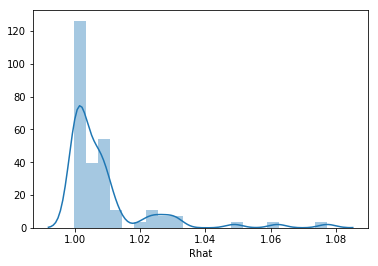## Plot posterior estimates of parameters¶

We can use plot_coefs to summarize posterior estimates of parameters.

In this basic pem_survival_model, we estimate a parameter for baseline hazard for each observed timepoint which is then adjusted for the duration of the timepoint. For consistency, the baseline values are normalized to the unit time given in the input data. This allows us to compare hazard estimates across timepoints without having to know the duration of a timepoint. (in general, the duration-adjusted hazard paramters are suffixed with _raw whereas those which are unit-normalized do not have a suffix).

In this model, the baseline hazard is parameterized by two components -- there is an overall mean across all timepoints (log_baseline_mu) and some variance per timepoint (log_baseline_tp). The degree of variance is estimated from the data as log_baseline_sigma. All components have weak default priors. See the stan code above for details.

In this case, the model estimates a minimal degree of variance across timepoints, which is good given that the simulated data assumed a constant hazard over time.

In :
survivalstan.utils.plot_coefs([testfit], element='baseline')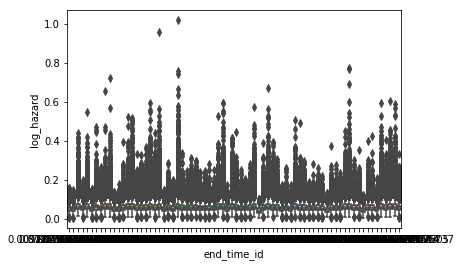We can also summarize the posterior estimates for our beta coefficients. This is actually the default behavior of plot_coefs. Here we hope to see the posterior estimates of beta coefficients include the value we used for our simulation (0.5).

In :
survivalstan.utils.plot_coefs([testfit])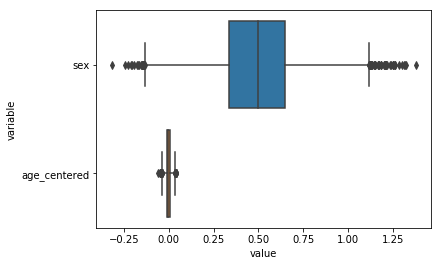## Posterior predictive checking¶

Finally, survivalstan provides some utilities for posterior predictive checking.

The goal of posterior-predictive checking is to compare the uncertainty of model predictions to observed values.

We are not doing true out-of-sample predictions, but we are able to sanity-check our model's calibration. We expect approximately 5% of observed values to fall outside of their corresponding 95% posterior-predicted intervals.

By default, survivalstan's plot_pp_survival method will plot whiskers at the 2.5th and 97.5th percentile values, corresponding to 95% predicted intervals.

In :
survivalstan.utils.plot_pp_survival([testfit], fill=False)
survivalstan.utils.plot_observed_survival(df=d, event_col='event', time_col='t', color='green', label='observed')
plt.legend()

Out:
<matplotlib.legend.Legend at 0x7efd0e2ffcc0>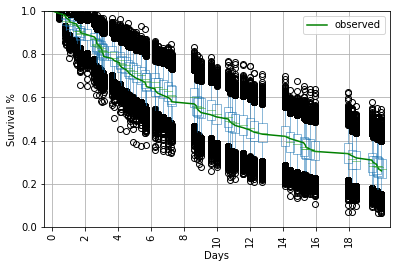We can also summarize and plot survival by our covariates of interest, provided they are included in the original dataframe provided to fit_stan_survival_model.

In :
survivalstan.utils.plot_pp_survival([testfit], by='sex')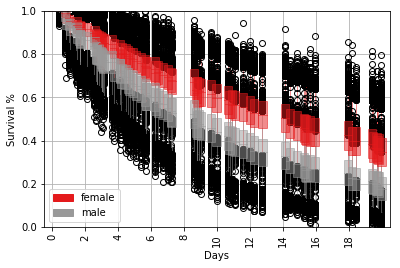This plot can also be customized by a variety of aesthetic elements

In :
survivalstan.utils.plot_pp_survival([testfit], by='sex', pal=['red', 'blue'])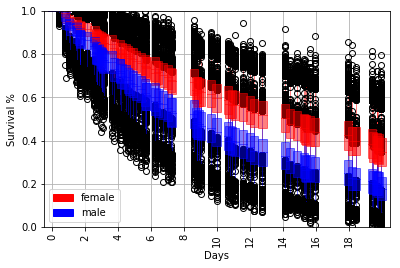## Building up the plot semi-manually, for more customization¶

We can also access the utility methods within survivalstan.utils to more or less produce the same plot. This sequence is intended to both illustrate how the above-described plot was constructed, and expose some of the functionality in a more concrete fashion.

Probably the most useful element is being able to summarize & return posterior-predicted values to begin with:

In :
ppsurv = survivalstan.utils.prep_pp_survival_data([testfit], by='sex')


Here are what these data look like:

In :
ppsurv.head()

Out:
iter model_cohort sex level_3 event_time survival
0 0 test model female 0 0.000000 1.000000
1 0 test model female 1 0.791192 1.000000
2 0 test model female 2 1.467963 0.975762
3 0 test model female 3 2.125944 0.920641
4 0 test model female 4 3.641794 0.911259

(Note that this itself is a summary of the posterior draws returned by survivalstan.utils.prep_pp_data. In this case, the survival stats are summarized by values of ['iter', 'model_cohort', by].

We can then call out to survivalstan.utils._plot_pp_survival_data to construct the plot. In this case, we overlay the posterior predicted intervals with observed values.

In :
subplot = plt.subplots(1, 1)
survivalstan.utils._plot_pp_survival_data(ppsurv.query('sex == "male"').copy(),
subplot=subplot, color='blue', alpha=0.5)
survivalstan.utils._plot_pp_survival_data(ppsurv.query('sex == "female"').copy(),
subplot=subplot, color='red', alpha=0.5)
survivalstan.utils.plot_observed_survival(df=d[d['sex']=='female'], event_col='event', time_col='t',
color='red', label='female')
survivalstan.utils.plot_observed_survival(df=d[d['sex']=='male'], event_col='event', time_col='t',
color='blue', label='male')
plt.legend()

Out:
<matplotlib.legend.Legend at 0x7efd0e2ca358>